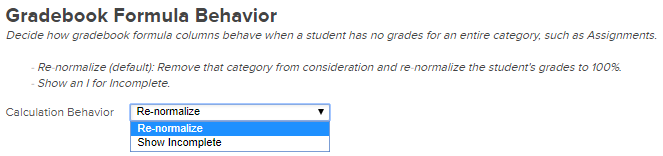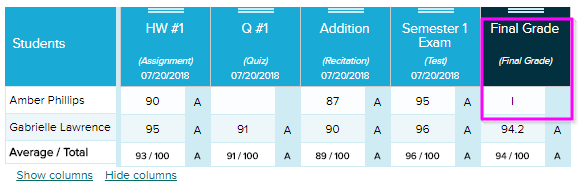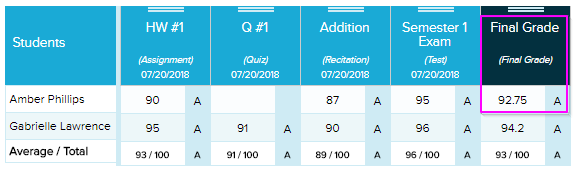# Calculating Final Grades with Incomplete Category

In Quickschools, the school administrators can decide how the Gradebook will treat a case when a student does not have grades for a specific column or category. This can be configured on the Grading Setup screen, under the section Gradebook Formula Behavior.In this example, we have the Final Grade column with the weight formula 50% Test, 10% Recitation, 20% Quiz, 20% Assignment, for a total of 100%.Set the Gradebook Formula Behavior to Show Incomplete if you want the formula to populate an “I” instead of a number. In the illustration below, the student is missing a grade in the category Quiz, which is 20% of the required weight factor to compute the Final Grade.Otherwise, set it to Re-normalize if you want the system to calculate the formula as though you never asked to include the “unfulfilled” category to begin with, instead of showing Incomplete. The system will then divide the grade to the total available weights, in this case 80, then computes the percentage to get 100 (See below).

Computation: ((90 x 0.20) + (87 x 0.10) + (95 x 0.50)) / 80 x 100 = 92.75If you’re ever concerned that a formula isn’t calculating accurately, please let us know. It’s always a good idea to verify the calculations on your end, either by hand or on an Excel sheet, to see if the result of the formula still seems incorrect. And if you do see a discrepancy, definitely contact QuickSchools so we can look into the issue.